Disclaimer: This is an example of a student written essay.

Any opinions, findings, conclusions or recommendations expressed in this material are those of the authors and do not necessarily reflect the views of UKEssays.com.

# Secure Barcode Authentication using Genetic Algorithm

 ✅ Paper Type: Free Essay ✅ Subject: Computer Science ✅ Wordcount: 4377 words ✅ Published: 3rd Apr 2018

• Dr. Poornima G. Naik
• Mr. Girish R. Naik

Abstract Genetic Algorithm (GA) is an invaluable tool for solving optimization problems due to its robustness. It does not break even if the inputs are changed slightly or in the presence of a reasonable noise. GA offers significant benefits over other optimization techniques in searching a large state space or n-dimensional surface. In todays information age information sharing and transfer has increased exponentially. With the popularization of Internet and exponential increase in e-commerce transactions security has become an inevitable and an integral part of any e-commerce application. Data integrity, confidentiality, authenticity, non-repudiation have gained tremendous importance and have become important components of information security. In this paper we have made an attempt to exploit the randomness involved in crossover and mutation processes of GA for generating a barcode for authentication process. The number of crossover points and number of mutation points is fixed and cannot be altered by the user. In the current work we have employed a single crossover point and two mutation points. We have used Code-39 and Code-128 encoding techniques for generating a barcode. The barcode data comprises of 12 randomly generated decimal digits. Each decimal digit is represented using 4 bits. Hence the length of the barcode data is 36 bits. The randomly generated data is transformed into encoded form by applying crossover, mutation and XOR operations before generating a bar code. The randomness together with encoding makes the password robust and hard to track. Finally, the algorithm is implemented in Java and applied for authentication of employee data in a hypothetical organization. The methodology is general and can be applied to any task where authentication is required.

Index Terms Genetic Algorithm, Cross-over, Mutation, Barcode, Encoding.

The paper is organized as follows. The first section gives an introduction to Genetic Algorithm under the heading of Introduction. Section II covers the literature survey and the current scenario of application of soft computing in implementing security. Section III focuses on the proposed method of barcode generation using Genetic Algorithm. Section IV covers implementation of the algorithm in Java. Finally, Section V is devoted for conclusion and scope for future enhancements.

I. Introduction

Genetic algorithms (GA) are adaptive heuristic search algorithms based on the evolutionary ideas of natural selection and genetics . They are based on the principle of Darwinian idea of survival of the fittest and natural genetics.

1. Genetic Algorithm

Generally, a Genetic Algorithm consists of three basic operations.

• Selection
• Crossover
• Mutation

The first step consists of searching individuals for reproduction. In our problem, we have selected two vectors of 16 bytes each as parents for reproduction. Since the problem is of encryption, there is no special preference given to any particular selection method. All the vectors are selected sequentially based on their order of appearance in a text file.

Cross-over is the process of taking two parents and producing from them a child. In an optimization problem, crossover operator is applied to the mating pool with the hope that it creates a better offspring. For the problem under consideration, crossover is taken as one of the steps in producing a decrypted vector. We have employed four-point crossover method. In the case of optimization problem, selecting more than four crossover points will result in the disruption of building blocks whereas in the case of encryption larger the disruption better is the algorithm which makes it robust and difficult to break.

If you need assistance with writing your essay, our professional essay writing service is here to help!

After crossover, the vectors are subject to mutation. In optimization problem, mutation prevents the algorithm from being trapped in a local minimum. Mutation plays the role of recovering the lost genetic matter as well for randomly distributed genetic information. In encryption problem, mutation is employed for inducing disorder into the vector. It introduces a new genetic structure in the population by randomly modifying some of the building blocks and maintains diversity into the population. We have employed flipping method, in which for a character 1 in mutation chromosome, the corresponding character b in the parent chromosome is flipped from b to (9-b) and corresponding child chromosome is produced. In the following example 1 occurs at

two random places of mutation chromosome, the corresponding characters in parent chromosomes are flipped and the child chromosomes are generated.

1. Structure of Code128 Bar Code

Barcodes are made up of a series of lines that vary in width and correspond to various numeric, alphanumeric, or multicode configurations which can then be read in by a laser barcode scanner.Code 128 is a very effective, high-density symbology which permits the encoding of alphanumeric data. It includes verification protection both via a checksum digit and byte parity checking. This symbology has been widely implemented in many applications where a relatively large amount of data must be encoded in a relatively small amount of space. Itsspecific structure also allows numeric data to be encoded at, effectively, double-density. A Code 128 barcode consists of a leading “quiet zone”, one of three start codes, the data itself, a check character, a stop character, and a trailing quiet zone as shown in Fig. 1. The Code 128 data is encoded in strips of bars and spaces. The sequences of zeros or ones simply appear as thicker bars or spaces. The checksum is included in the barcode, and is a digit that verifies that the data just read in was correct. The checksum digit is based on a modulo 103 calculation based on the weighted sum of the values of each of the digits in the message that is being encoded, including the start character.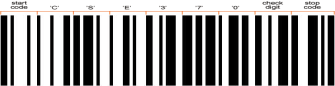Fig. 1. Code-128 Barcode

Similar structure exists for Code-39 Barcode.

ii Literature survey

In literature to date, many GA based encryption algorithms have been proposed. A. Tragha et.al  have describe a new symmetric block cipher system namely, ICIGA (Improved Cryptographic Inspired by Genetic Algorithm) which generates a session key in a random process. The block size and key length are variables and can be fixed by the end user in the beginning of the cipher process. ICIGA is an enhancement of the system GIC (Genetic Algorithm inspired Cryptography) . There are various proposed methods for image encryption such as quad tree approach, cellular automata [4, 5]. There are wide applications of GA in solving non-linear optimization problems in various domains [6,7]. But very few papers exist which exploit the randomness in the algorithm for implementation of security. Chaos theory and entropy have large application in secure data communication and the desired disorder is provided by inherent nature of genetic algorithm [8, 10]. Mohammad SazzadulHoque et.al  have presented an intrusion detection system by applying GA to efficiently detect various types of network intrusions. They have used evolutionary theory to filter the traffic data and thus reduce the complexity . There are several papers related to IDS all of which use GA in deriving classification rules [13, 15]. But to the best of our knowledge very few papers exist which exploit randomness in generating barcode for authentication purpose.

III Proposed Method

Fig. 2. Application Architecture

We have used Code-39 and Code-128 encoding techniques for generating a barcode. The barcode data comprises of 12 randomly generated decimal digits. Each decimal digit is represented using 4 bits. Hence the length of the barcode data is 36 bits.The randomly generated data is transformed into encoded form by applying crossover, mutation and XOR operations before generating a bar code. The application architecture is shown in Fig. 2.

 Data Encoding Barcode Generation Physical File
1. Pseudocode

The pseudo code for barcode generation process using GA is depicted in Fig 3.

Step 1 : Generate a 12 digit random number and store it in a vector.

Step 2 : Each decimal digit in step 1 can be represented using 4 binary digits. Hence the total number of binary digits required to represent the data is 4 x 12 = 48 bits. Generate a hash H, by repeating digits 0 and 1 (if the digit is > 8) and 0 and 0, otherwise, required number of times.

Step 3 : Perform the XOR operation between the data and a 48-bit hash computed above.

Step 4 : Split the vector into two vectors of size six each.

Step 5 : Compute 10’s complement of each digit.

Step 6 : Perform the crossover operation at the midpoint.

Step 7 : Perform the mutation at the extreme positions of the vector. The mutation operation consists of flipping the digit from its original value to its complement.

Step 8 : Combine the vectors to reconstruct a 12-digit vector.

Step 9 : Perform the XOR operation between the data and a 48-bit hash computed above.

Step 10 : Use the 12-digit number generated above to generate a barcode in code-128 fromat.

Step 11 : End

Fig 3 Pseudo code for barcode generation using GA

1. Mathematical Formulation.

Let the original vector be represented by VOriginal. Let H be the hash constructed as follows.

H= ∑’ Hi where 1 <= i <= 12 and ∑’ is the string concatenation operator.

Hi = 0000, for i = 8 or 9

= 0101, otherwise.

H is the generated hash of length 48 bits.

Compute the hash of VOriginal as shown below:

VOriginal θ H = VHash

Split the hash into two vectors of size six each. Let the two parts be represented by, V1Hash and V2Hash, respectively.

VHash = V1Hash + V2Hash

Compute 10’s complement of each digit. Let the two parts be represented by ( V1Hash)Ì and (V2Hash )Ì, respectively.

Perform the crossover operation at the midpoint. Let the two new parts now be represented by C( V1Hash )Ì and C(V2Hash ), respectively, where C is the crossover operator.

Perform the mutation at the extreme positions of the vector. Let the two parts now be represented by MC( V1Hash )Ì and MC(V2Hash ) Ì, respectively, where M is the crossover operator. Combine the vectors to reconstruct a 12-digit vector.

Perform the XOR operation between the data and a 48-bit hash, H computed above to generate a final vector. Let it be VTransformed. We get,

VTransformed = [ MC( V1Hash )Ì + MC(V2Hash ) Ì] θ H (1)

Decoding Vector into original Vector

Perform XOR operation between H and VTransformed given by equ(1) to get, [ MC( V1Hash )Ì + MC(V2Hash ) Ì].

Split the hash into two vectors of size six each. Let the two parts be represented by, MC( V1Hash )Ì and MC(V2Hash ) Ì respectively.

Perform reverse mutation operation and then reverse cross0ver operation on two individual parts to get, ( V1Hash)Ì and (V2Hash ) Ì, respectively.

Take 10’s complement of each digit in the two vectors to get, ( V1Hash) and (V2Hash ), respectively.

Combine the two vectors to get VHash, where

VHash =VOriginal θ H

Perform XOR operation between H and VHashto get the original vector.

The entire process of generating the barcode is illustrated below with the help of an example.

Step 1: Generate a 12 digit random number and store it in a vector. Let the number be represented by

 8 6 9 3 1 8 9 8 3 9 5 3

Step 2 : Generate Hash H as shown below.

 0 5 0 5 5 0 0 0 5 0 5 5

Step 3 : Perform the XOR operation between the data and a 48-bit hash computed above.

 8 3 9 6 4 8 9 8 6 9 0 6

Step 4 : Split the vector into two vectors of size six each.

 8 3 9 6 4 8

and

 9 8 6 9 0 6

Step 5 : Compute 10’s complement of each digit.

 1 6 0 4 5 1

and

 0 1 3 0 9 3

Step 6 : Perform the crossover operation at the midpoint.

 0 9 3 4 5 1

and

 0 1 3 1 6 0

Step 7 : Perform the mutation at the extreme positions of the vector.

 9 9 3 4 5 8
 9 1 3 1 6 9

Step 8 : Combine the vectors to reconstruct a 12-digit vector.

 9 9 3 4 5 8 9 1 3 1 6 9

Step 9 :Generate Hash H as shown below..

 0 0 5 5 5 0 0 5 5 5 5 0

Step 10 : Perform the XOR operation between the data and a 48-bit hash computed above

 9 9 6 1 0 8 9 4 6 4 3 9

Step 11 : Use the 12-digit number generated above to generate a barcode in code-128 fromat.

CODE128- 996108946439.

1. Decoding the barcode

Step 1: Extract the rightmost 12 digits from the barcode.

 9 9 6 1 0 8 9 4 6 4 3 9

Step 2 : Generate a hash as shown below:

 0 0 5 5 5 0 0 5 5 5 5 0

Step 3 : Perform the XOR operation between the data and a 48-bit hash computed above

 9 9 3 4 5 8 9 1 3 1 6 9

Step 4 : Split the vector into two vectors of size six each.

 9 9 3 4 5 8
 9 1 3 1 6 9

Step 5 : Perform reverse mutation at the extreme positions of the vector.

 0 9 3 4 5 1

and

 0 1 3 1 6 0

Step 6 : Perform the crossover operation at the midpoint.

 1 6 0 4 5 1

and

 0 1 3 0 9 3

Step 7 : Compute 10’s complement of each digit.

 8 3 9 6 4 8

and

 9 8 6 9 0 6

Step 8 : Combine the vectors to reconstruct a 12-digit vector.

 8 3 9 6 4 8 9 8 6 9 0 6

Step 9 : Generate a hash as shown below:

 0 5 0 5 5 0 0 0 5 0 0 5

Step 10 : Perform the XOR operation between the data and a 48-bit hash computed above

 8 6 9 3 1 8 9 8 3 9 5 3

which represents the original vector

iv implementation in JAVA

The model proposed above is implemented in Java using MS Access as backend and Swing for GUI development. JDBC Type-I driver is used. The structure of the Barcode table used in the implementation is as follows :

 Column Name Column Type Description Name Text Stores User Name of an Employee Barcode Text Stores encoded barcode data. ID Text Stores unique identification for an Employee.

The following figures 4.1 to 4.4 show the output windows generated by Barcode tool developed in Java.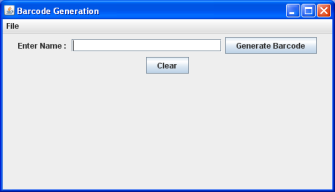Fig. 4.1 Java Barcode Generation Tool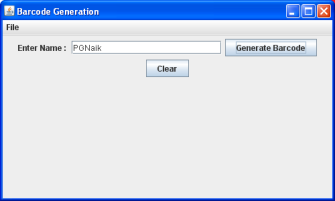Fig. 4.2 Generation of Barcode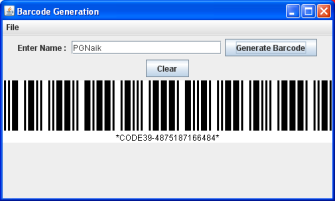Fig. 4.3 Barcode generated in Code-39 Format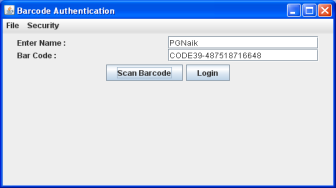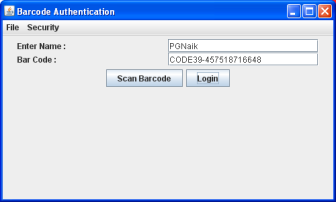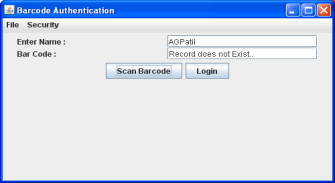Fig. 4.4 Barcode Authentication Process.

V.CONCLUSION AND SCOPE FOR FUTURE WORK

In this paper we have proposed a model for barcode generation based on genetic algorithm and is implemented in Java for authentication of employees in a hypothetical organization. The password is encrypted by applying crossover, mutation and XOR operations and is difficult to track. This model provides a unique security layer on top of existing barcode security layer which makes the password more robust and difficult to break. Even if the database is hacked, the password cannot be stolen because the relationship between barcode and ID is not known. The model can be employed in situations where authentication is of prime significance and can be used for secure transmission of limited data such as credit card number. It provides a cheaper solution to RFID for authentication. Due to the symmetry in the operations involved and symmetry of XOR operation, the coding and encoding processes are reversible. Our future work consists of interfacing the software with barcode scanner and study of various coding techniques with reference to their applicability.

## References

1. David. E. Goldberg, “Genetic Algorithms in Search, Optimization, and Machine Learning”, Pearson Education, 1989, ISBN-13: 978-020115767.
2. X. F. Liao, S. Y.Lai and Q. Zhou. Signal Processing. 90 (2010) 2714–2722.
3. H. Cheng and X. Li. IEEE Transactions on Signal Processive. 48 (8) (2000) 2439–2451.
4. O. Lafe. Engineering Applications of Artificial Intelligence. 10 (6) (1998) 581–591.
5. R. J. Chen and J. L. Lai. Pattern Recognition. 40 (2007) 1621–1631
6. Dr.Poornima G. Naik, Girish R. Naik, Application of Genetic Algorithm to Mass Production Line for Productivity Improvement, International Journal of Latest Trends in Engineering and Technology (IJLTET) Special Issue – IDEAS-2013 ISSN:2278-621X.
7. S. Li, G. Chen and X. Zheng. Multimedia security handbook. LLC, Boca Raton, FL, USA: CRC Press; (2004) [chapter 4].
8. Y. Mao and G. Chen. Handbook of computational geometry for pattern recognition, computer vision, neural computing and robotics. Springer; (2003).
9. H. S. Kwok, W. K. S. Tang, Chaos Solitons and Fractals, (2007) 1518–1529.
10. Mohammad SazzadulHoque, Md. Abdul Mukit and Md. Abu NaserBikas,An Implementation of Intrusion Detection System Using Genetic Algorithm, International Journal of Network Security & Its Applications (IJNSA), Vol.4, No.2, March 2012
11. L.M.R.J Lobo, Suhas B. Chavan, Use of Genetic Algorithm in Network Security, International Journal of Computer Applications (0975 – 8887)Volume 53– No.8, September 2012
12. W. Lu, I. Traore, “Detecting New Forms of Network Intrusion Using Genetic Programming”. Computational Intelligence, vol. 20, pp. 3, Blackwell Publishing, Malden, pp. 475-494, 2004.
13. M. M. Pillai, J. H. P. Eloff, H. S. Venter, “An Approach to Implement a Network Intrusion Detection System using Genetic Algorithms”, Proceedings of SAICSIT, pp:221-228, 2004.
14. S. M. Bridges, R. B. Vaughn, “Fuzzy Data Mining And Genetic Algorithms Applied To Intrusion Detection”, Proceedings of 12th Annual Canadian Information Technology Security Symposium, pp. 109-122, 2000.
15. M. Middlemiss, G. Dick, “Feature selection of intrusion detection data using a hybrid geneticalgorithm/KNN approach”, Design and application of hybrid intelligent systems, IOS Press Amsterdam, pp.519-527, 2003.

View all

## DMCA / Removal Request

If you are the original writer of this essay and no longer wish to have your work published on UKEssays.com then please:

Related Services

Prices from

\$USD152

Approximate costs for:

• 1000 words
• 7 day deliveryHumanity University

Dedicated to your worth and value as a human being!

Related Lectures